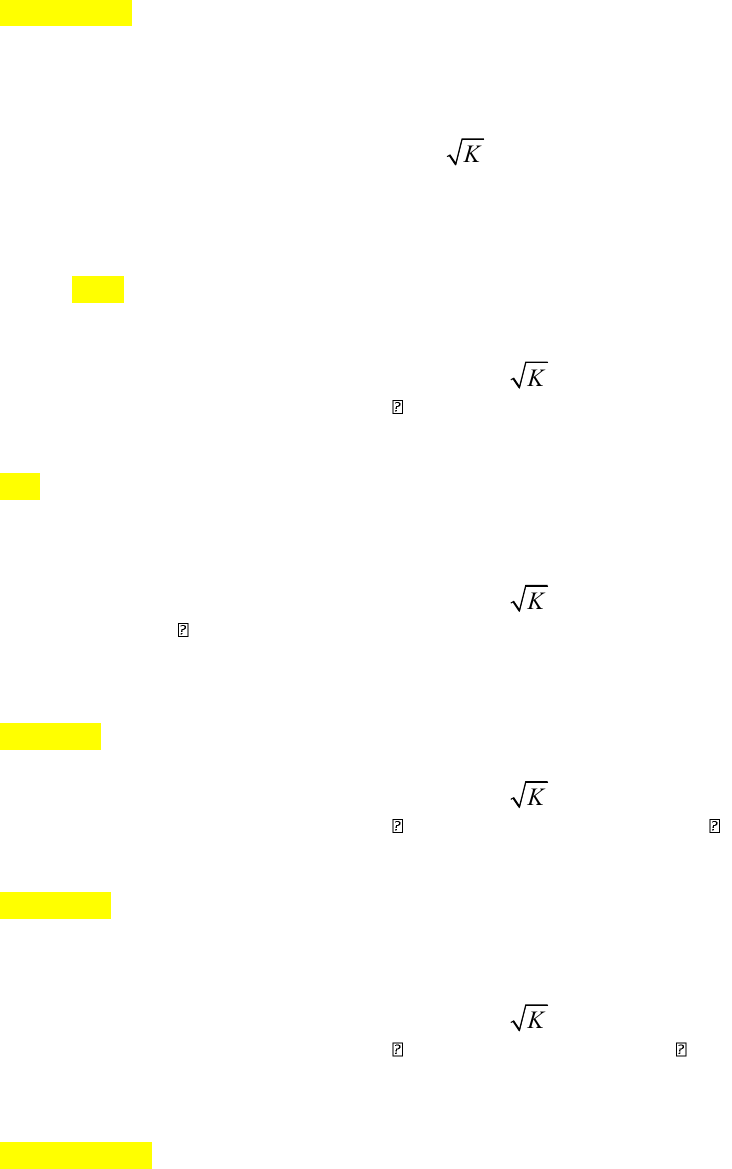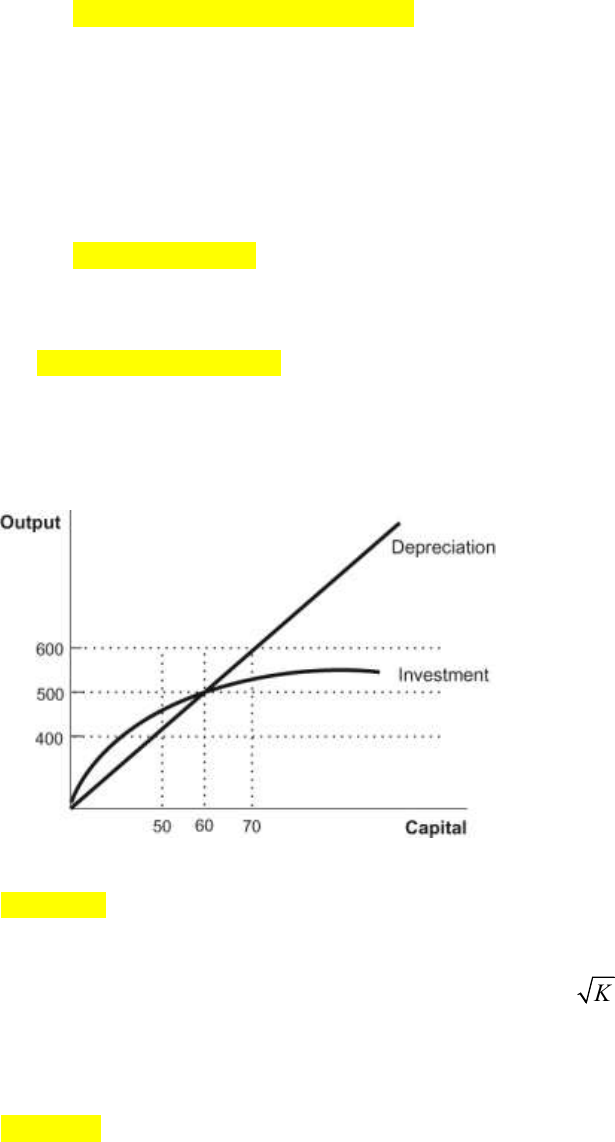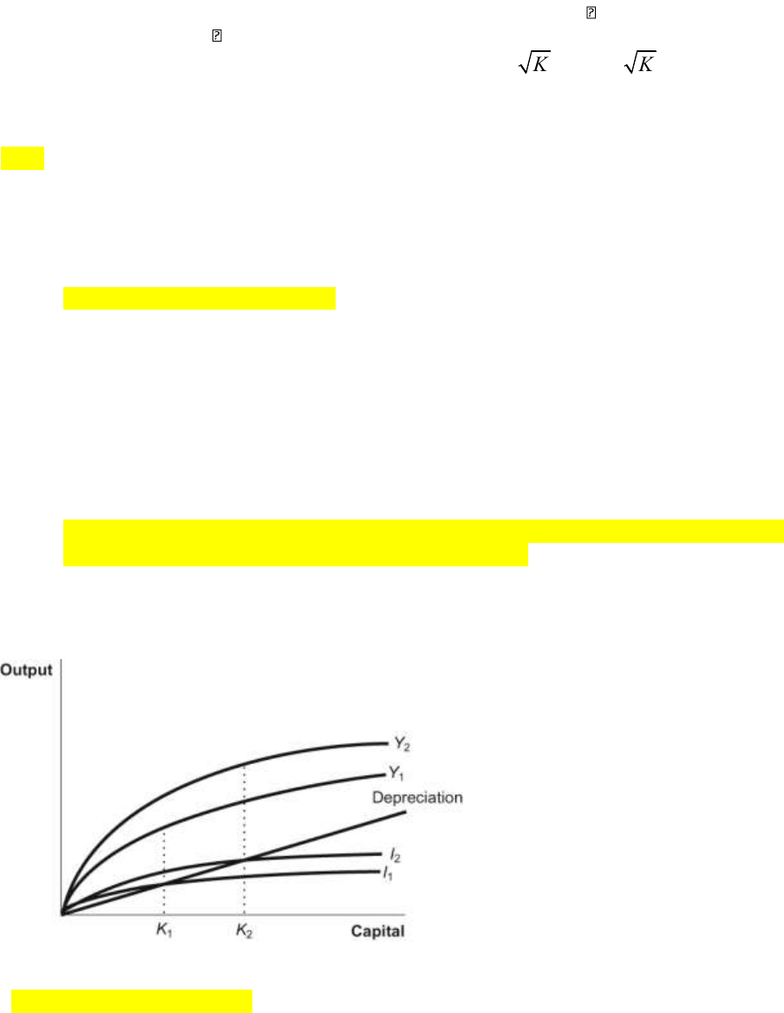# ECON 2001.01 Lecture Notes - Lecture 17: Production Function, Capital Accumulation, Finished Good

151 views15 pages
School
Ohio State University
Department
Economics
Course
ECON 2001.01
ProfessorECON 2002.01
Practice Questions for Exam II
1. The Solow growth model features _____ returns to physical capital.
A) diminishing
B) constant
C) increasing
D) no
2. . An economy with production function Y = F(K) =
K
that has 400 units of capital will produce
_____ units of output.
A) 1,600
B) 400
C) 40
D) 20
3. Imagine an economy with production function Y = F(K) =
K
and 400 units of capital. If the
fraction of output invested in new capital is = 0.2, how much new capital will be created in the
next period?
A) 2
B) 4
C) 6
D) 8
4. Imagine an economy with production function Y = F(K) =
K
and 400 units of capital. If the
depreciation rate is = 0.05, how much capital will deteriorate in the next period?
A) 4 units
B) 8 units
C) 16 units
D) 20 units
5. Imagine an economy with production function Y = F(K) =
K
and 400 units of capital. If the
fraction of output invested in new capital is = 0.2 and the depreciation rate is = 0.05, how
much total capital will be available in the next period?
A) 380 units
B) 384 units
C) 400 units
D) 404 units
6. Imagine an economy with production function Y = F(K) =
K
and 400 units of capital. If the
fraction of output invested in new capital is = 0.2, the depreciation rate is = 0.05, and the
economy starts with output of 20, what does the Solow model predict will happen to output in
the long run?
A) It will remain at 20.
B) It will decline.
C) It will increase.
Unlock document

This preview shows pages 1-3 of the document.
Unlock all 15 pages and 3 million more documents.ECON 2002.01
D) It will increase for a time and then return to 20.
7. In the Solow model, if the first unit of capital increases output by one unit, then the second unit
of capital will cause total output to:
A) increase, but by less than one unit.
B) increase by more than one unit.
C) remain the same.
D) decrease.
8. Germany and Japan's rapid growth following the end of World War II is an example of:
A) indirect growth.
B) cutting-edge growth.
C) growth due to increases in natural resources.
D) catch-up growth.
9. In a steady state, the level of investment:
A) exceeds depreciation.
B) is equal to depreciation.
C) is less than depreciation.
D) could be equal to, greater than, or less than depreciation.
10. (Figure: Depreciation and Investment) According to this diagram of the Solow model, the steady-
state level of the capital stock is:
A) 40 units.
B) 50 units.
C) 60 units.
D) 70 units.
11. If an economy described by the production function Y =
K
has 30% of output invested in new
capital and 6% of the capital stock depreciated each year, what is the steady-state level of
capital?
A) 3.24 units
B) 9 units
C) 25 units
D) 36 units
Unlock document

This preview shows pages 1-3 of the document.
Unlock all 15 pages and 3 million more documents.ECON 2002.01
12. A country in a steady state invests 50% of its output in new capital ( = 0.5) and depreciates 5%
of its capital stock ( = 0.05). With a capital stock of 100 units, labor remains constant. Because
of technological innovation, production improves from Y =
K
to Y = 2
K
. What is the new
A) 10
B) 20
C) 40
D) 80
13. Good institutions tend to:
A) decrease the rate of investment.
B) leave the rate of investment unchanged.
C) increase the rate of investment.
D) have an ambiguous effect on investment.
14. Conditional convergence refers to the tendency for:
A) poorer countries to grow faster than richer countries, but only if they receive sufficient
foreign investment.
B) richer countries to grow faster than poorer countries given similar steady-state capital
stocks, so the poor countries never catch up with the rich countries.
C) poorer countries to grow faster than richer countries given similar steady-state capital
stocks, but the poor countries will never catch up with the rich countries.
D) countries with similar steady-state levels of output to grow faster when they're poor than
when they're rich until their per capita GDP levels converge.
15. (Figure: The Solow Model) Which of the following events would NOT shift the production
function from Y1 to Y2 in the accompanying diagram of the Solow model?
A) increases in productivity
B) better ideas
C) physical capital accumulation